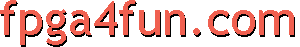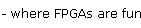## SPI 2 - A simple implementation

##### ARM processor

To get an opportunity to test our newly acquired SPI knowledge, we use a Saxo-L board. It has an ARM7 processor (LPC2138) and a Cyclone FPGA (EP1C3), connected by a SPI bus.

The ARM is used as a SPI master, while the FPGA is used as a SPI slave. The Saxo-L ARM processor has actually two SPI interfaces, one called SPI0, and a more advanced one called SPI1/SSP. They are both equally easy to use. We are using SPI1/SSP on Saxo-L, as it is pre-wired on the board.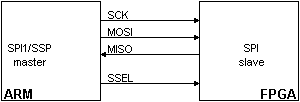##### SPI master - C ARM code

Using SSP is just a matter of initializing a few registers, and then writing/reading data to send/receive automatically.

```void main(void)
{
// initialize SSP
SSP0CPSR = 0x02;  // SSP max speed
SSP0CR0 = 0x07;  // SSP max speed, 8 bits
SSP0CR1 = 0x02;  // SSP master mode
PINSEL1 = 0x2A8;  // SSP mode for pins P0.17 to P0.20

while(1)
{
// send two bytes
SSP0DR = 0x55;  // one nice thing about the SSP is that it has a 8-words deep FIFO
SSP0DR = 0x54;  // so here we write the data to be sent without worrying

// now wait until both bytes are sent
while(!(SSP0SR & 0x01));

// now we can read the two bytes received... and do anything with them
int data1 = SSP0DR;
int data2 = SSP0DR;
// ...
}
}
```
##### SPI slave - HDL FPGA code

Now for the SPI slave in the FPGA.

Since the SPI bus is typically much slower than the FPGA operating clock speed, we choose to over-sample the SPI bus using the FPGA clock. That makes the slave code slightly more complicated, but has the advantage of having the SPI logic run in the FPGA clock domain, which will make things easier afterwards.

First the module declaration.

```module SPI_slave(clk, SCK, MOSI, MISO, SSEL, LED);
input clk;

input SCK, SSEL, MOSI;
output MISO;

output LED;
```

Note that we have "clk" (the FPGA clock) and an LED output... a nice little debug tool. "clk" needs to be faster than the SPI bus. Saxo-L has a default clock of 24MHz, which works fine here.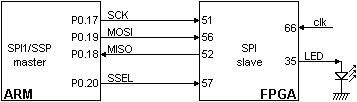We sample/synchronize the SPI signals (SCK, SSEL and MOSI) using the FPGA clock and shift registers.

```// sync SCK to the FPGA clock using a 3-bits shift register
reg [2:0] SCKr;  always @(posedge clk) SCKr <= {SCKr[1:0], SCK};
wire SCK_risingedge = (SCKr[2:1]==2'b01);  // now we can detect SCK rising edges
wire SCK_fallingedge = (SCKr[2:1]==2'b10);  // and falling edges

// same thing for SSEL
reg [2:0] SSELr;  always @(posedge clk) SSELr <= {SSELr[1:0], SSEL};
wire SSEL_active = ~SSELr;  // SSEL is active low
wire SSEL_startmessage = (SSELr[2:1]==2'b10);  // message starts at falling edge
wire SSEL_endmessage = (SSELr[2:1]==2'b01);  // message stops at rising edge

// and for MOSI
reg [1:0] MOSIr;  always @(posedge clk) MOSIr <= {MOSIr, MOSI};
wire MOSI_data = MOSIr;
```

Now receiving data from the SPI bus is easy.

```// we handle SPI in 8-bits format, so we need a 3 bits counter to count the bits as they come in
reg [2:0] bitcnt;

always @(posedge clk)
begin
if(~SSEL_active)
bitcnt <= 3'b000;
else
if(SCK_risingedge)
begin
bitcnt <= bitcnt + 3'b001;

// implement a shift-left register (since we receive the data MSB first)
end
end

always @(posedge clk) byte_received <= SSEL_active && SCK_risingedge && (bitcnt==3'b111);

// we use the LSB of the data received to control an LED
reg LED;
```

Finally the transmission part.

```reg [7:0] byte_data_sent;

reg [7:0] cnt;
always @(posedge clk) if(SSEL_startmessage) cnt<=cnt+8'h1;  // count the messages

always @(posedge clk)
if(SSEL_active)
begin
if(SSEL_startmessage)
byte_data_sent <= cnt;  // first byte sent in a message is the message count
else
if(SCK_fallingedge)
begin
if(bitcnt==3'b000)
byte_data_sent <= 8'h00;  // after that, we send 0s
else
byte_data_sent <= {byte_data_sent[6:0], 1'b0};
end
end

assign MISO = byte_data_sent;  // send MSB first
// we assume that there is only one slave on the SPI bus
// so we don't bother with a tri-state buffer for MISO
// otherwise we would need to tri-state MISO when SSEL is inactive

endmodule
```

We have established communication between the ARM and the FPGA!

##### Running the code

As we step through the ARM code, we can see the LED changing state, and the data returned by the FPGA.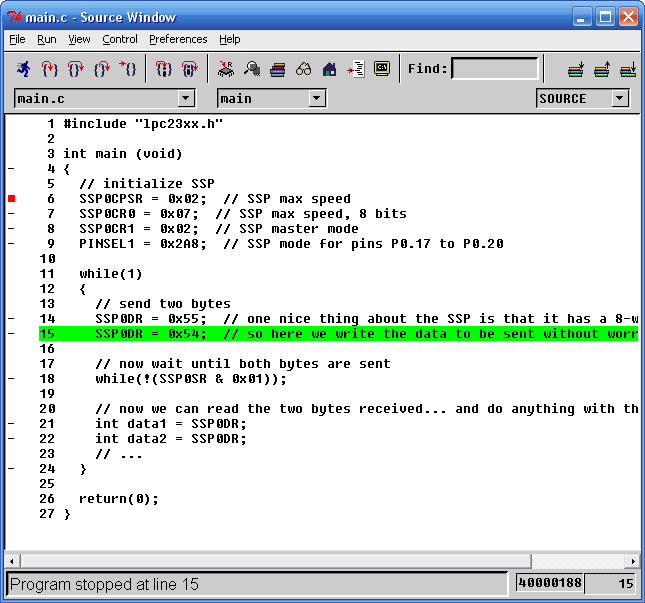Now let's see if we can do useful things with SPI.